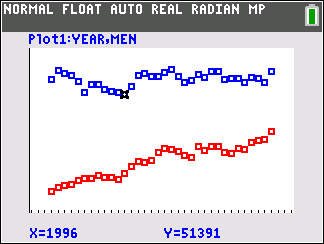•• ##### Device
•TI-84 Plus C Silver Edition
•TI-84 Plus CE
• ##### Software

TI-SmartView™ CE

# Mathematical Modeling: Modeling Median Incomes

by Texas Instruments#### Objectives

Students will be able to:

• Model a contextual situation mathematically and use the model to make a prediction
• Represent two quantitative variables on a scatter plot, and describe how the variables are related.
• Find and interpret linear equations to model relationships between two quantitative variables;
• Use proportional relationships to solve real-world and mathematical problems